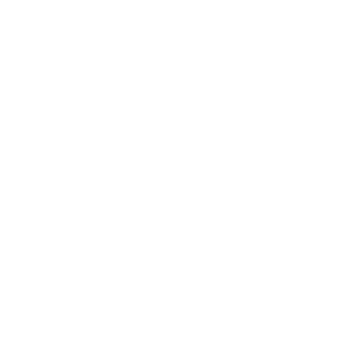# C++ Functions

The C++ functions are user-defined named block of code that performs a specific task. The function takes parameter or no parameters, it may return a value or not return anything. Every function has a type that indicates the return type of that function.

The main() is a function in C++ program and there could only be one main function. The default return type of main function is integer. A user-defined function can call other functions except main function.

## How to use functions in C++ program?

There are three things to remember while using functions in C++ programs.

1. Declare the function
2. Define the function
3. Call the function in your program.

### Function Declaration

A function declaration is a way to declare return type, number and data type of parameters. There are two places you can declare your function. First, you can declare your function globally outside of main function. Second, you can declare the function inside of main function.

For example,

void addition( int a, int b);
int multiplication( int x, int y);

In the first example, the return type is void which means the function is not going to return any value. The function name is addition and it has two integer parameters.

The second example has a return type of int, the function name is multiplication and there are two integer parameters. The parameters are called formal parameters.

### Function Definition

The function definition contains the actual code for the function. The function definition must match the function declaration, otherwise, it will result in compiler error.

For example,

void addition( int a, int b)
{
//Variable declaration and initialization
int total = 0;
total = a + b;
// Print the results
cout << "Total =" << " " << total << endl;
}

The function definition has code to perform the addition in the above program. It matches the function declaration we did previously. Note that the function does not return the value, but prints the output.

### Calling Function

The function is used several times during the execution of a program. The main function or other user-defined function can call a specific function to perform a task.

For example,

void main()
{
// Variable and function declaration
int a, int b;
void addition( int a, int b);
// Calling a function
}

The main function calls the function addition with two actual parameters. The actual parameters does not need type declaration, the variable name is sufficient. The function name does not need a return type because it is already declared at the beginning.

### Actual Parameters vs. Formal Parameters

The formal parameter is used as a dummy parameter. Its purpose is to declare the type and number of parameter being used in the function.

The actual parameter is the variable value that get passed on to the function. The names used for actual parameter must match with variable names.

The formal parameter does not need variable names, even data type is enough to declare or define the function.

For example,

float square (float , float , double);

The above code contains three parameters for the function. A variable name is not used for any parameters, but still it a valid declaration.

In the next lesson, we will learn about function types, and variable scopes.